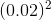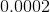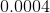## Example Questions

### Example Question #1 : How To Square A Decimal

Find the square of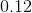.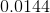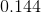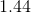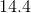Explanation:

Squaring a decimal is identical to multiplying decimals.

Drop the decimal, multiply the number by its self, and then add up the decimal points in the original problem and put it back into your answer.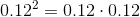(note: 4 decimal places)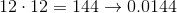### Example Question #1 : How To Square A Decimal

What is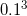?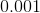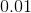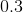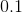Explanation:

You can solve this on your calculator, or think about it as a fraction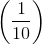to make the problem easier to do by hand.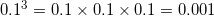.

### Example Question #1 : How To Square A Decimal

If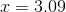and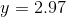, what is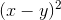equal to?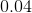Explanation:

First, we must figure out what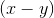is equal to.

We do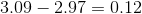.

Now to find out what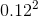is equal to, we look at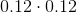.

We move our decimal points over so that we are dealing with only whole numbers. This gives us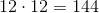.

Finally, we count how many spaces we moved our decimals in total, and we move the decimal in our answer back that many spaces. To get fromtowe moved our decimal two spaces to the right. Because we did this for each of thevalues, our total spaces we moved the decimal wasto the right.

Therefore, we must takeand move the decimalplaces to the left. This gives us.

### Example Question #2 : How To Square A Decimal

Evaluate the following: